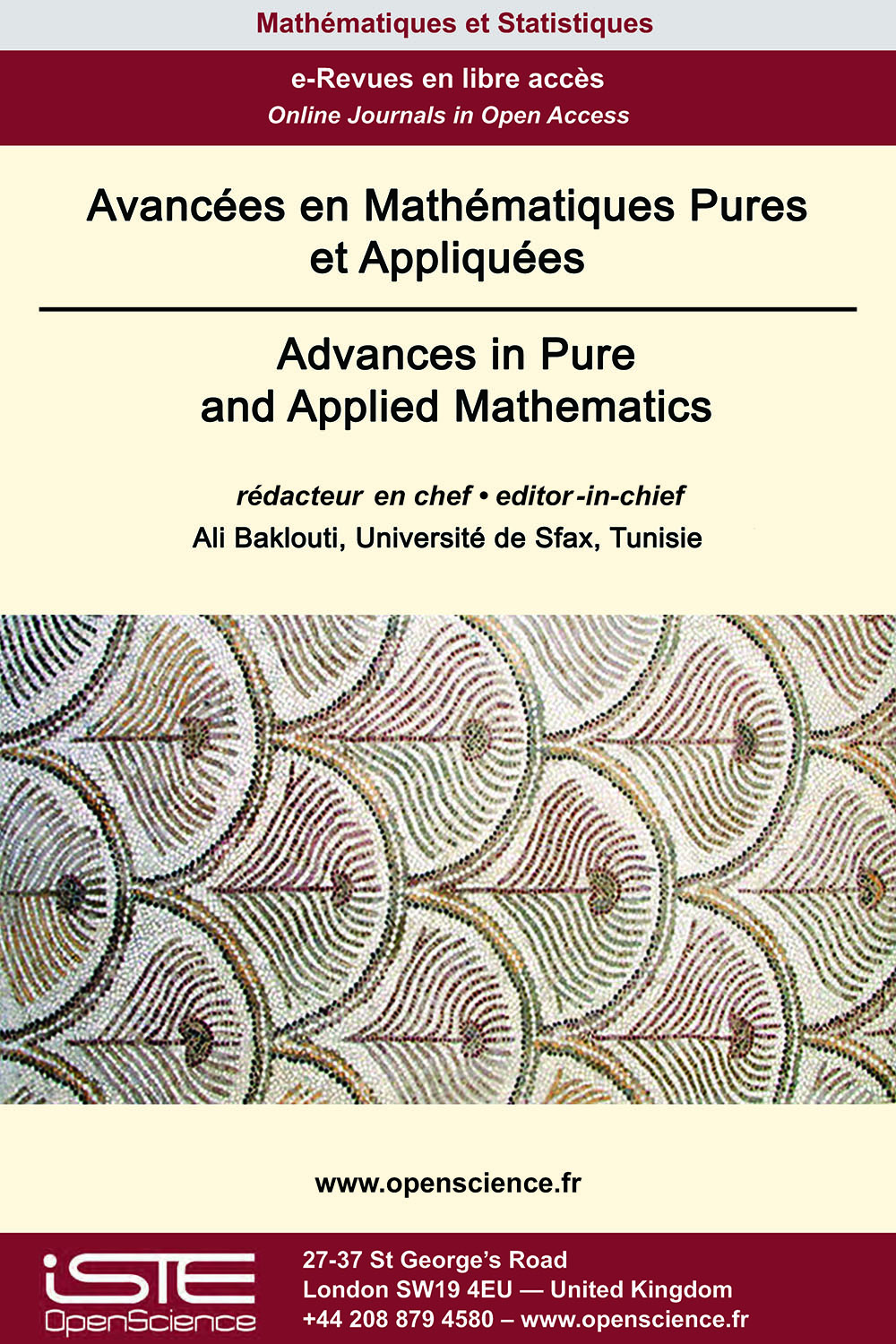# Vol 13 - À paraître

## Articles parus

[FORTHCOMING] Extension des operations semistar

Let RT be an extension of integral domains and ∗ be a semistar operation stable of finite type on R. We define a semistar operation ∗1 on T in the following way : for each nonzero T-submodule E of the quotient field K1 of T, let E∗1 = ∪ {E :K1 JT | J ∈ $\mathcal{F}$∗}, where K1 denotes the quotient field of T and $\mathcal{F}$∗ the localizing system associated to ∗. In this paper we investigate the basic properties of ∗1. Moreover, we show that the map $\varphi$ which associates to a semistar operation ∗ stable and of finite type on R, the semistar operation ∗1 is continuous. Furthermore, we give sufficient conditions for $\varphi$ to be a homeomorphism.

[FORTHCOMING] Existence globale de solutions du système sphérique-symétrique de Einstein-Vlasov-Maxwell

We prove that the initial value problem with small data for the asymptotically flat spherically symmetric Einstein-Vlasov-Maxwell system admits the global in time solution in the case of the non zero shift vector. This result extends the one already known for chargeless case.### Autres numéros :

2020

Volume 20- 11

Numéro 1 (Mai 2020)
Numéro 2 (Septembre 2020)

2021

Volume 21- 12

Numéro 1 (Janvier 2021)
Numéro 2 (Mai 2021)
Numéro spécial : AUS-ICMS 2020
Numéro 3 (Septembre 2021)

2022

Volume 22- 13

À paraître

Numéro 1 (Janvier 2022)
Numéro 2 (Mars 2022)
Numéro 3 (Juin 2022)
Numéro 4 (Septembre 2022)

2023

Volume 23- 14

Numéro 1 (Janvier 2023)